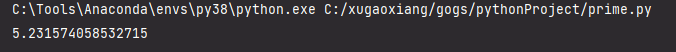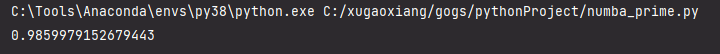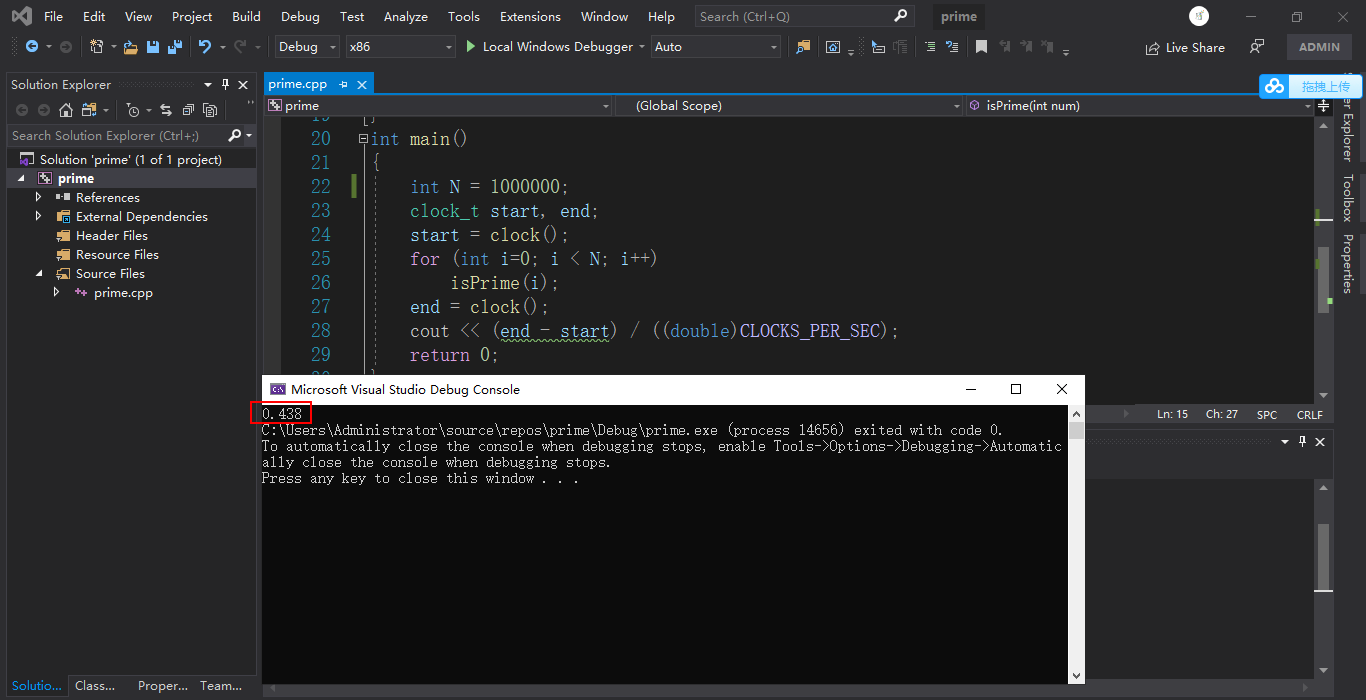# numba加速python

## 前言

`Python`是一门应用非常广泛的高级语言，但是，长久以来，`Python`的运行速度一直被人诟病，相比`c/c++``java``c#``javascript`等一众高级编程语言，完全没有优势。

## 示例

``````import math
import time

def is_prime(num):
if num == 2:
return True

if num <= 1 or not num % 2:
return False

# 从3开始，到int(根号num)+1，步长是2，如3,5,7 ...
for i in range(3, int(math.sqrt(num)) + 1, 2):
if not num % i:
return False

return True

def run_program(N):
for i in range(N):
is_prime(i)

if __name__ == '__main__':
N = 1000000
start = time.time()
run_program(N)
end = time.time()
print(end - start)
``````## 改进

`numba`就是这么一款工具，它是`python`的即时编译器(`just-in-time compiler`)，它使用`LLVM``python`代码翻译成机器码，特别是在使用`numpy`数组以及循环操作上，效果最佳。

`numba`的使用比较简单，我们不需要更换`python`的解释器，只需要将`numba`的装饰器写在`python`方法上，当这个带有`numba`装饰器的方法被调用时，就会被`just-in-time`即时编译为机器代码，然后执行。

``````pip install numba

conda install numba``````

``````import math
import time
from numba import njit

@njit(fastmath=True, cache=True)
def is_prime(num):
if num == 2:
return True
if num <= 1 or not num % 2:
return False

for i in range(3, int(math.sqrt(num)) + 1, 2):
if not num % i:
return False
return True

@njit(fastmath=True, cache=True)
def run_program(N):
for i in range(N):
is_prime(i)

if __name__ == '__main__':
N = 10000000
start = time.time()
run_program(N)
end = time.time()
print(end - start)
````````````#include <iostream>
#include <cmath>
#include <time.h>

using namespace std;

bool isPrime(int num)
{
if (num == 2)
return true;
if (num <= 1 || num % 2 == 0)
return false;
double sqrt_num = sqrt(double(num));
for (int i = 3; i <= sqrt_num; i += 2) {
if (num % i == 0)
return false;
}
return true;
}

int main()
{
int N = 1000000;
clock_t start, end;
start = clock();
for (int i=0; i < N; i++)
isPrime(i);
end = clock();
cout << (end - start) / ((double)CLOCKS_PER_SEC);
return 0;
}``````